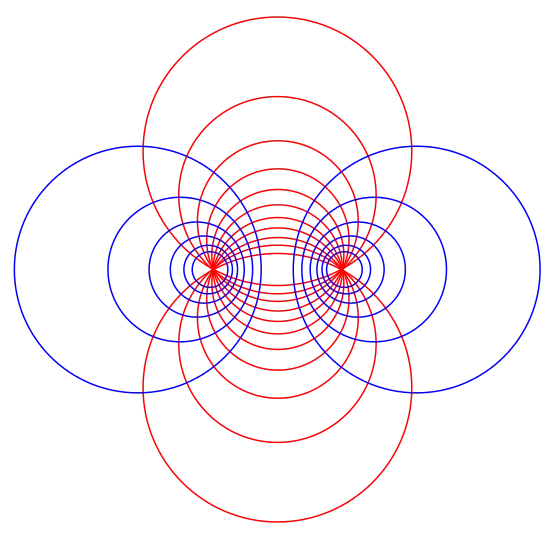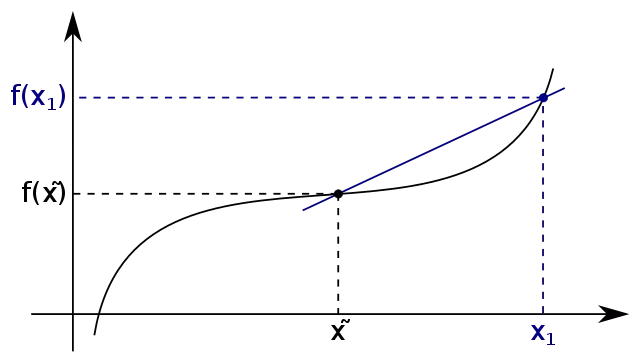# Tag differentiation IIT JEE

## Differential Equations Class 12 notes | IIT-JEE study materialSo, in calculus we are starting the topic of differential equations, we will be seeing what does a differential equation exactly means, what are partial differential equations, the significance of differential equations in various disciplines is very vast, we will…

## Methods of Differentiation | Concept Booster IIT-JEE Class 12 notesMethods of Differentiation Here we will learn methods of Differentiation, We had already studied the two meanings of derivative(differentiation) of a function which is the geometrical and physical meanings. Since we know what does the differentiation of a function means…